# 栈和队列-题解-方法

2021-09-11 11:13 141 查看

# 用栈实现队列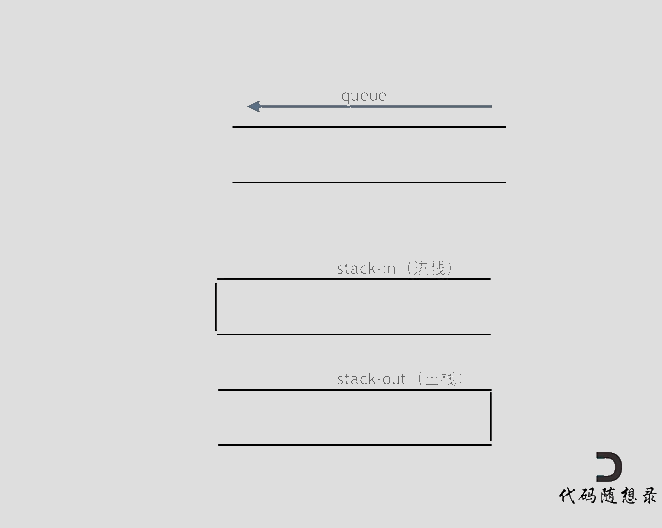```class MyQueue {

Stack<Integer> stack1;
Stack<Integer> stack2;

/** Initialize your data structure here. */
public MyQueue() {
stack1 = new Stack<>(); // 负责进栈
stack2 = new Stack<>(); // 负责出栈
}

/** Push element x to the back of queue. */
public void push(int x) {
stack1.push(x);
}

/** Removes the element from in front of queue and returns that element. */
public int pop() {
dumpStack1();
return stack2.pop();
}

/** Get the front element. */
public int peek() {
dumpStack1();
return stack2.peek();
}

/** Returns whether the queue is empty. */
public boolean empty() {
return stack1.isEmpty() && stack2.isEmpty();
}

// 如果stack2为空，那么将stack1中的元素全部放到stack2中
private void dumpStack1(){
if (stack2.isEmpty()){
while (!stack1.isEmpty()){
stack2.push(stack1.pop());
}
}
}
}

/**
* Your MyQueue object will be instantiated and called as such:
* MyQueue obj = new MyQueue();
* obj.push(x);
* int param_2 = obj.pop();
* int param_3 = obj.peek();
* boolean param_4 = obj.empty();
*/```

# 用队列实现栈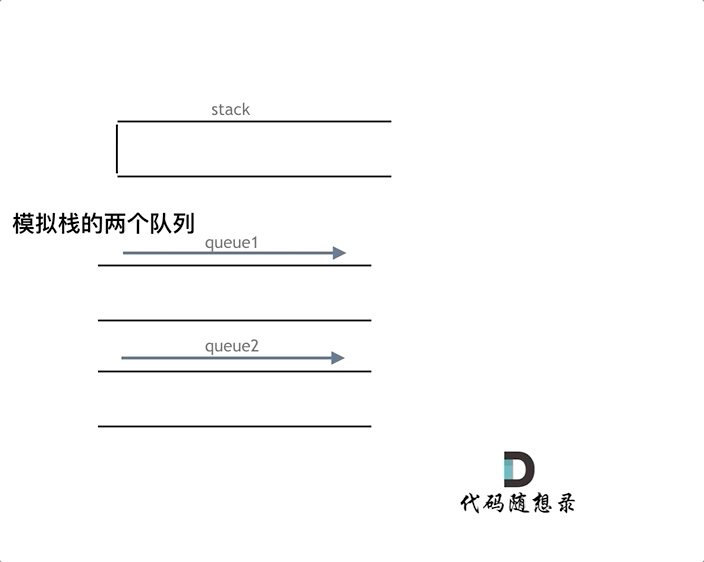```class MyStack {

Queue<Integer> queue1; // 和栈中保持一样元素的队列
Queue<Integer> queue2; // 辅助队列

/** Initialize your data structure here. */
public MyStack() {
}

/** Push element x onto stack. */
public void push(int x) {
queue2.offer(x); // 先放在辅助队列中
while (!queue1.isEmpty()){
queue2.offer(queue1.poll());
}
Queue<Integer> queueTemp;
queueTemp = queue1;
queue1 = queue2;
queue2 = queueTemp; // 最后交换queue1和queue2，将元素都放到queue1中
}

/** Removes the element on top of the stack and returns that element. */
public int pop() {
return queue1.poll(); // 因为queue1中的元素和栈中的保持一致，所以这个和下面两个的操作只看queue1即可
}

/** Get the top element. */
public int top() {
return queue1.peek();
}

/** Returns whether the stack is empty. */
public boolean empty() {
return queue1.isEmpty();
}
}```

```class MyStack {
// Deque 接口继承了 Queue 接口
Deque<Integer> que1; // 和栈中保持一样元素的队列
Deque<Integer> que2; // 辅助队列
/** Initialize your data structure here. */
public MyStack() {
que1 = new ArrayDeque<>();
que2 = new ArrayDeque<>();
}

/** Push element x onto stack. */
public void push(int x) {
}

/** Removes the element on top of the stack and returns that element. */
public int pop() {
int size = que1.size();
size--;
// 将 que1 导入 que2 ，但留下最后一个值
while (size-- > 0) {
que1.pollFirst();
}

int res = que1.pollFirst();
// 将 que2 对象的引用赋给了 que1 ，此时 que1，que2 指向同一个队列
que1 = que2;
// 如果直接操作 que2，que1 也会受到影响，所以为 que2 分配一个新的空间
que2 = new ArrayDeque<>();
return res;
}

/** Get the top element. */
public int top() {
return que1.peekLast();
}

/** Returns whether the stack is empty. */
public boolean empty() {
return que1.isEmpty();
}
}```

```class MyStack {
// Deque 接口继承了 Queue 接口
Deque<Integer> que1;
/** Initialize your data structure here. */
public MyStack() {
que1 = new ArrayDeque<>();
}

/** Push element x onto stack. */
public void push(int x) {
}

/** Removes the element on top of the stack and returns that element. */
public int pop() {
int size = que1.size();
size--;
// 将 que1 导入 que2 ，但留下最后一个值
while (size-- > 0) {
que1.pollFirst();
}

int res = que1.pollFirst();
return res;
}

/** Get the top element. */
public int top() {
return que1.peekLast();
}

/** Returns whether the stack is empty. */
public boolean empty() {
return que1.isEmpty();
}
}```

# 有效的括号

• 左括号必须用相同类型的右括号闭合。
• 左括号必须以正确的顺序闭合。
• 注意空字符串可被认为是有效字符串。

• 输入: "()"
• 输出: true
```class Solution {
public boolean isValid(String s) {
char ch;
for (int i = 0; i < s.length(); i++) {
ch = s.charAt(i);
//碰到左括号，就把相应的右括号入栈
if (ch == '(') {
deque.push(')');
}else if (ch == '{') {
deque.push('}');
}else if (ch == '[') {
deque.push(']');
} else if (deque.isEmpty() || deque.peek() != ch) {
return false;
}else {//如果是右括号判断是否和栈顶元素匹配
deque.pop();
}
}
//最后判断栈中元素是否匹配
return deque.isEmpty();
}
}```

# 删除字符串中的所有相邻重复项

• 输入："abbaca"
• 输出："ca"
• 解释：例如，在 "abbaca" 中，我们可以删除 "bb" 由于两字母相邻且相同，这是此时唯一可以执行删除操作的重复项。之后我们得到字符串 "aaca"，其中又只有 "aa" 可以执行重复项删除操作，所以最后的字符串为 "ca"。

• 1 <= S.length <= 20000
• S 仅由小写英文字母组成。

## 题外话

`Segmentation fault`
（当然不是所有的
`Segmentation fault`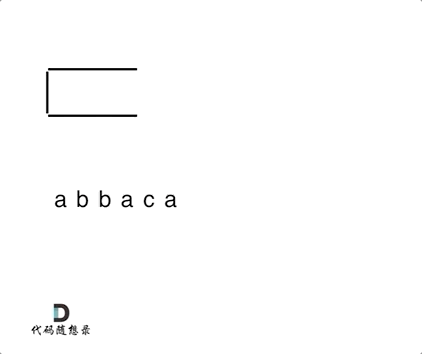```class Solution {
public String removeDuplicates(String S) {
ArrayDeque<Character> deque = new ArrayDeque<>();
char ch;
for (int i = 0; i < S.length(); i++) {
ch = S.charAt(i);
if (deque.isEmpty() || deque.peek() != ch) {
deque.push(ch);
} else {
deque.pop();
}
}
String str = "";
//剩余的元素即为不重复的元素
while (!deque.isEmpty()) {
str = deque.pop() + str;
}
return str;
}
}```

```class Solution {
public String removeDuplicates(String s) {
// 将 res 当做栈
StringBuffer res = new StringBuffer();
// top为 res 的长度
int top = -1;
for (int i = 0; i < s.length(); i++) {
char c = s.charAt(i);
// 当 top > 0,即栈中有字符时，当前字符如果和栈中字符相等，弹出栈顶字符，同时 top--
if (top >= 0 && res.charAt(top) == c) {
res.deleteCharAt(top);
top--;
// 否则，将该字符 入栈，同时top++
} else {
res.append(c);
top++;
}
}
return res.toString();
}
}```

```class Solution {
public String removeDuplicates(String s) {
char[] ch = s.toCharArray();
int fast = 0;
int slow = 0;
while(fast < s.length()){
// 直接用fast指针覆盖slow指针的值
ch[slow] = ch[fast];
// 遇到前后相同值的，就跳过，即slow指针后退一步，下次循环就可以直接被覆盖掉了
if(slow > 0 && ch[slow] == ch[slow - 1]){
slow--;
}else{
slow++;
}
fast++;
}
return new String(ch,0,slow);
}
}```

# 逆波兰表达式求值

• 输入: ["2", "1", "+", "3", " * "]
• 输出: 9
• 解释: 该算式转化为常见的中缀算术表达式为：((2 + 1) * 3) = 9

• 去掉括号后表达式无歧义，上式即便写成 1 2 + 3 4 + * 也可以依据次序计算出正确结果。
• 适合用栈操作运算：遇到数字则入栈；遇到算符则取出栈顶两个数字进行计算，并将结果压入栈中。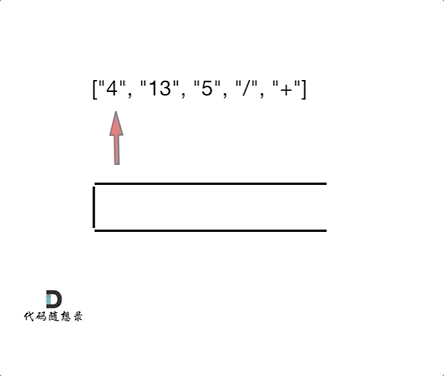```public class EvalRPN {

public int evalRPN(String[] tokens) {
for (String token : tokens) {
char c = token.charAt(0);
if (!isOpe(token)) {
} else if (c == '+') {
stack.push(stack.pop() + stack.pop());
} else if (c == '-') {
stack.push(- stack.pop() + stack.pop());
} else if (c == '*') {
stack.push( stack.pop() * stack.pop());
} else {
int num1 = stack.pop();
int num2 = stack.pop();
stack.push( num2/num1);
}
}
return stack.pop();
}
private boolean isOpe(String s) {
return s.length() == 1 && s.charAt(0) <'0' || s.charAt(0) >'9';
}
private int stoi(String s) {
return Integer.valueOf(s);
}

public static void main(String[] args) {
new EvalRPN().evalRPN(new String[] {"10","6","9","3","+","-11","*","/","*","17","+","5","+"});
}

}```

# 滑动窗口最大值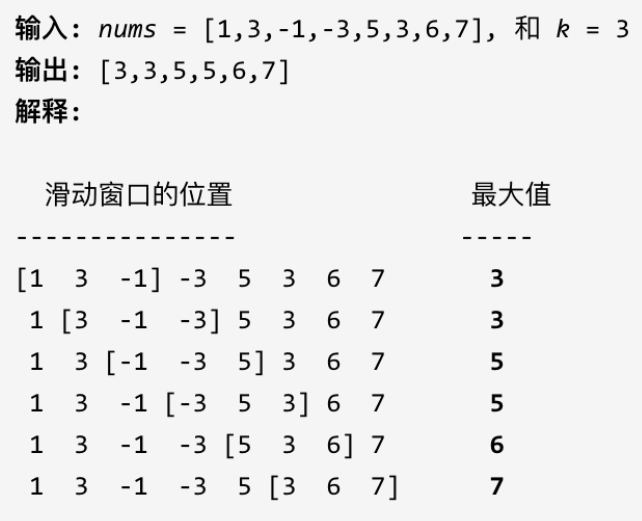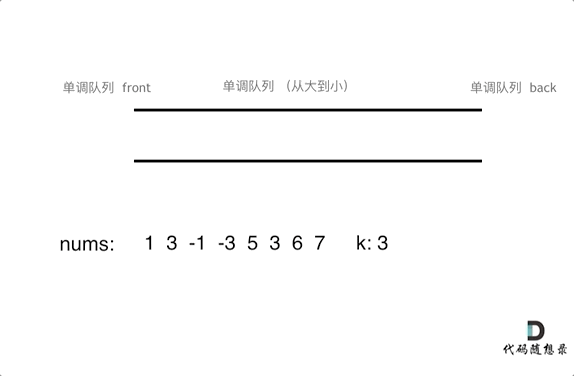```//解法一
//自定义数组
class MyQueue {
//弹出元素时，比较当前要弹出的数值是否等于队列出口的数值，如果相等则弹出
//同时判断队列当前是否为空
void poll(int val) {
if (!deque.isEmpty() && val == deque.peek()) {
deque.poll();
}
}
//添加元素时，如果要添加的元素大于入口处的元素，就将入口元素弹出
//保证队列元素单调递减
//比如此时队列元素3,1，2将要入队，比1大，所以1弹出，此时队列：3,2
while (!deque.isEmpty() && val > deque.getLast()) {
deque.removeLast();
}
}
//队列队顶元素始终为最大值
int peek() {
return deque.peek();
}
}

class Solution {
public int[] maxSlidingWindow(int[] nums, int k) {
if (nums.length == 1) {
return nums;
}
int len = nums.length - k + 1;
//存放结果元素的数组
int[] res = new int[len];
int num = 0;
//自定义队列
MyQueue myQueue = new MyQueue();
//先将前k的元素放入队列
for (int i = 0; i < k; i++) {
}
res[num++] = myQueue.peek();
for (int i = k; i < nums.length; i++) {
//滑动窗口移除最前面的元素，移除是判断该元素是否放入队列
myQueue.poll(nums[i - k]);
//滑动窗口加入最后面的元素
//记录对应的最大值
res[num++] = myQueue.peek();
}
return res;
}
}

//解法二
//利用双端队列手动实现单调队列
/**
* 用一个单调队列来存储对应的下标，每当窗口滑动的时候，直接取队列的头部指针对应的值放入结果集即可
* 单调队列类似 （tail -->） 3 --> 2 --> 1 --> 0 (--> head) (右边为头结点，元素存的是下标)
*/
class Solution {
public int[] maxSlidingWindow(int[] nums, int k) {
ArrayDeque<Integer> deque = new ArrayDeque<>();
int n = nums.length;
int[] res = new int[n - k + 1];
int idx = 0;
for(int i = 0; i < n; i++) {
// 根据题意，i为nums下标，是要在[i - k + 1, i] 中选到最大值，只需要保证两点
// 1.队列头结点需要在[i - k + 1, i]范围内，不符合则要弹出
while(!deque.isEmpty() && deque.peek() < i - k + 1){
deque.poll();
}
// 2.既然是单调，就要保证每次放进去的数字要比末尾的都大，否则也弹出
while(!deque.isEmpty() && nums[deque.peekLast()] < nums[i]) {
deque.pollLast();
}

deque.offer(i);

// 因为单调，当i增长到符合第一个k范围的时候，每滑动一步都将队列头节点放入结果就行了
if(i >= k - 1){
res[idx++] = nums[deque.peek()];
}
}
return res;
}
}```

# 前 K 个高频元素

• 输入: nums = [1,1,1,2,2,3], k = 2
• 输出: [1,2]

1. 要统计元素出现频率
2. 对频率排序
3. 找出前K个高频元素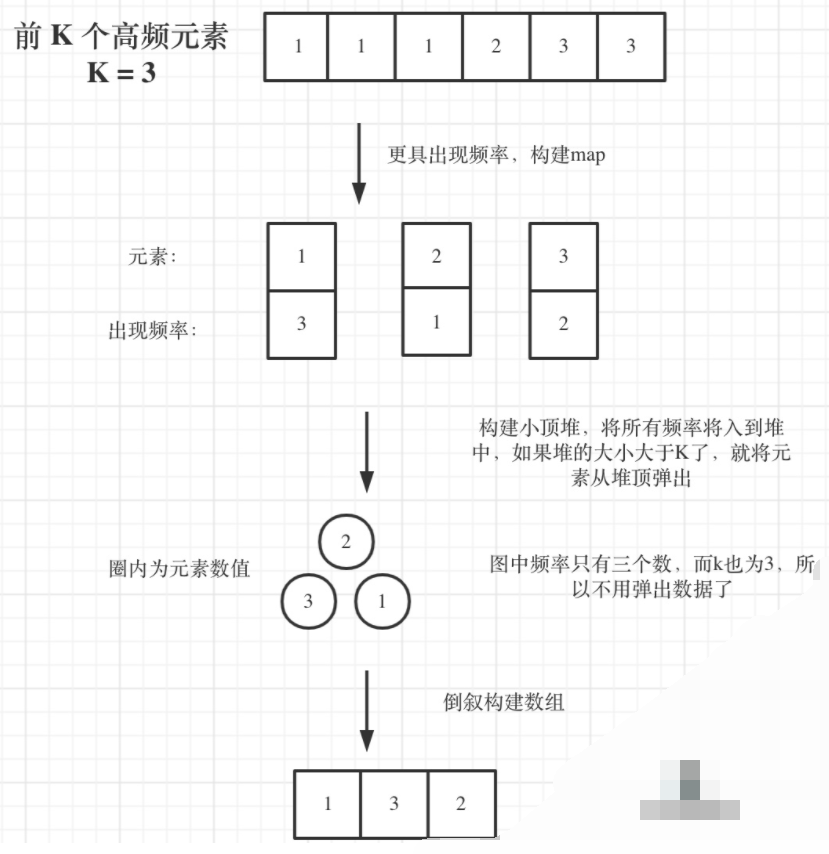```class Solution {
public int[] topKFrequent(int[] nums, int k) {
int[] result = new int[k];
HashMap<Integer, Integer> map = new HashMap<>();
for (int num : nums) {
map.put(num, map.getOrDefault(num, 0) + 1);
}

Set<Map.Entry<Integer, Integer>> entries = map.entrySet();
// 根据map的value值正序排，相当于一个小顶堆
PriorityQueue<Map.Entry<Integer, Integer>> queue = new PriorityQueue<>((o1, o2) -> o1.getValue() - o2.getValue());
for (Map.Entry<Integer, Integer> entry : entries) {
queue.offer(entry);
if (queue.size() > k) {
queue.poll();
}
}
for (int i = k - 1; i >= 0; i--) {
result[i] = queue.poll().getKey();
}
return result;
}
}```